# Lines and Slopes Example 1 Find the Slope

• Slides: 36Lines and Slopes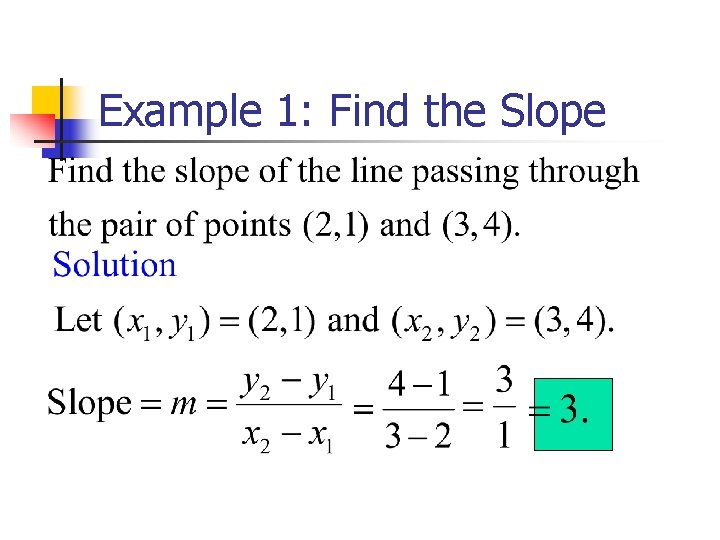Example 1: Find the Slope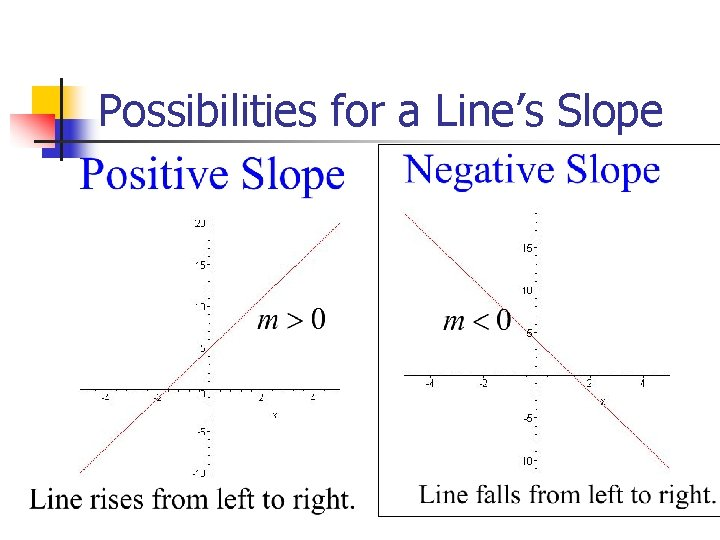Possibilities for a Line’s Slope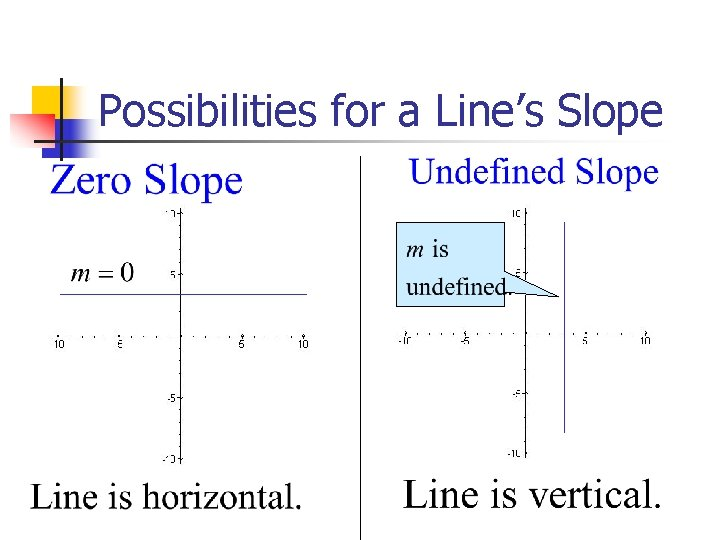Possibilities for a Line’s Slope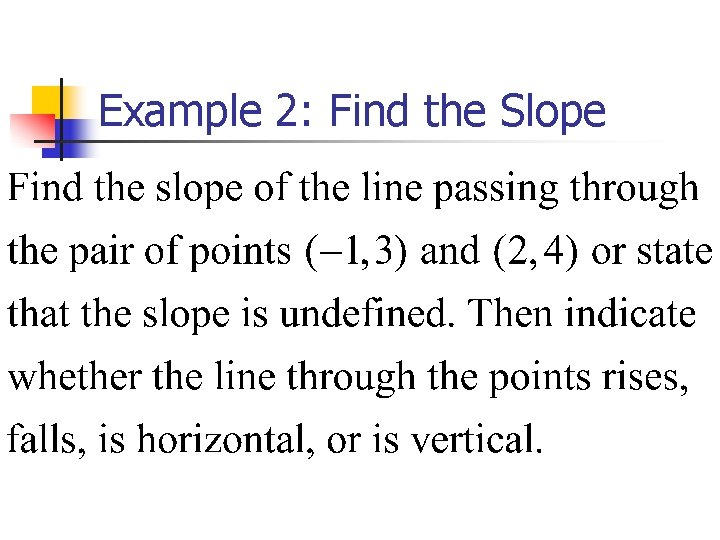Example 2: Find the SlopeSolution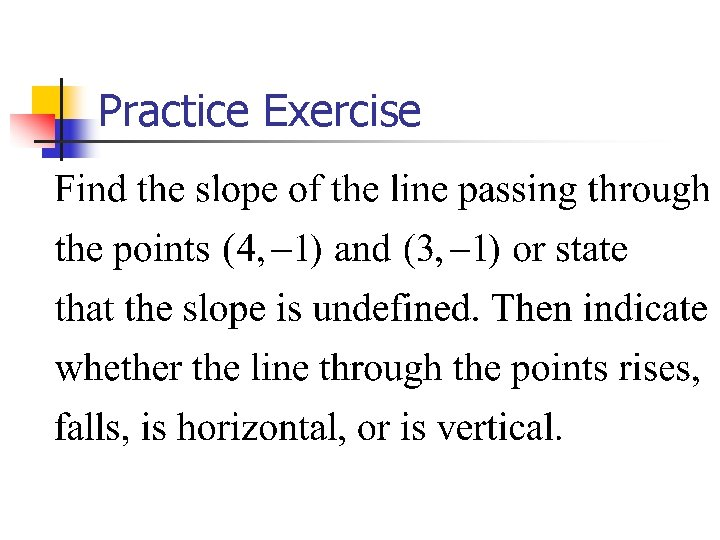Practice Exercise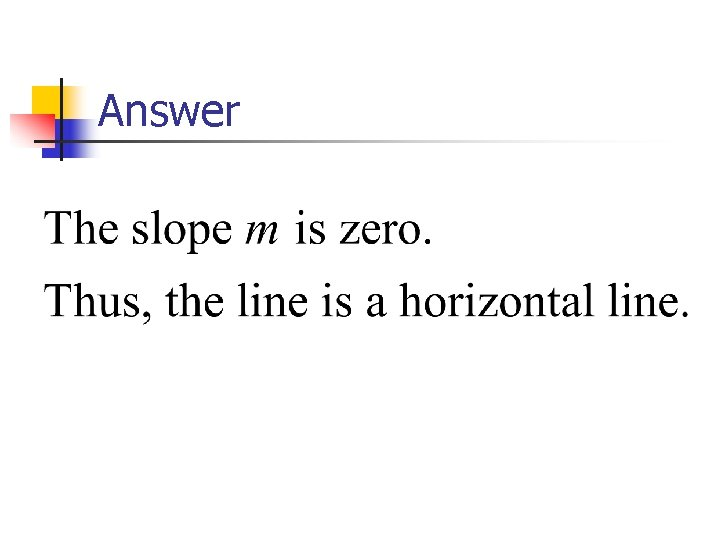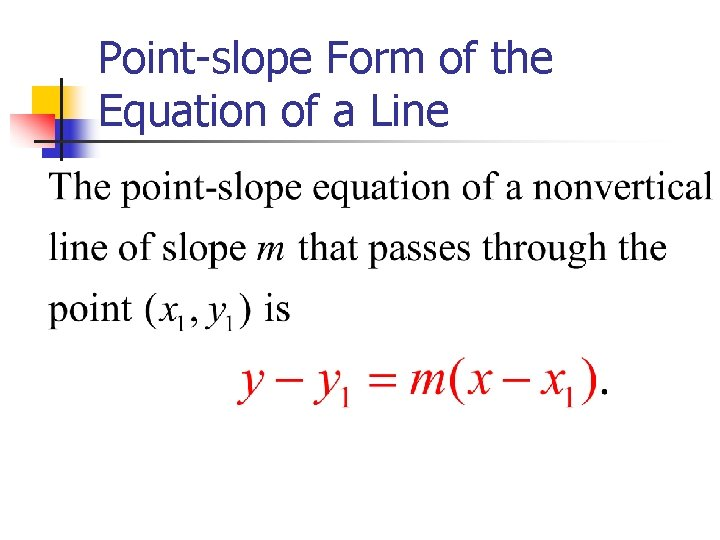Point-slope Form of the Equation of a LineExample 3: Writing the Point. Slope Equation of a Line Write the point-slope form of the equation of the line passing through (1, 3) with a slope of 4. Then solve the equation for y.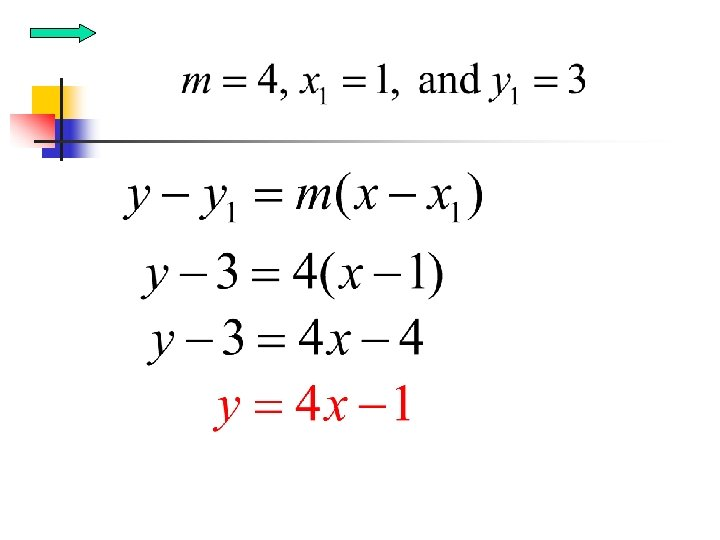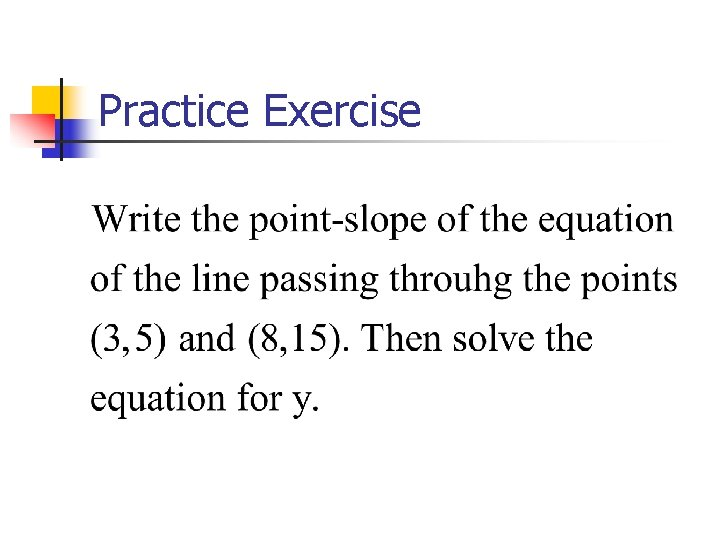Practice Exercise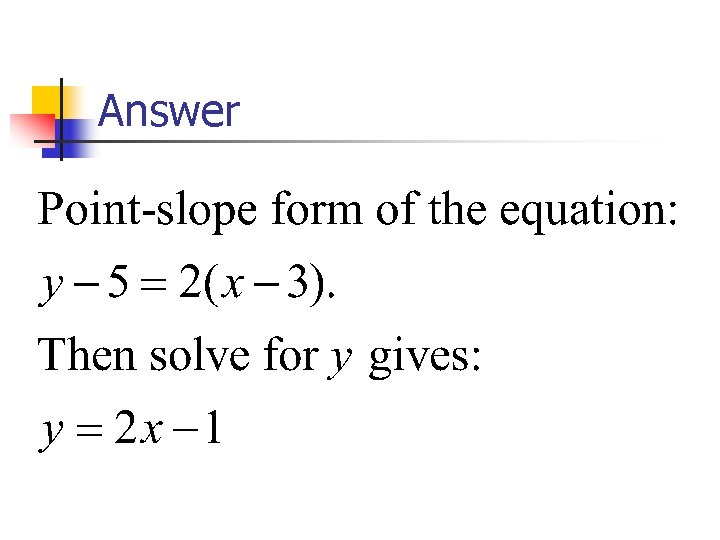Practice Exercises 1. Write the point-slope form of the equation of the line passing through (4, -1) with a slope of 8. Then solve the equation for y. 2. Write the point-slope form of the equation of the line passing through the points ( -2, 0) and (0, 2). Then solve the equation for y.Answers to Practice ExercisesThe Slope-Intercept Form of the Equation of a Line Y-intercept is b Slope is mGraphing y=mx+b Using the Slope and y-Intercept. Plot the y-intercept on the y-axis. This is the point (0, b). n Obtain a second point using the slope, m. Write m as a fraction, and use rise over run starting at the yintercept to plot this point n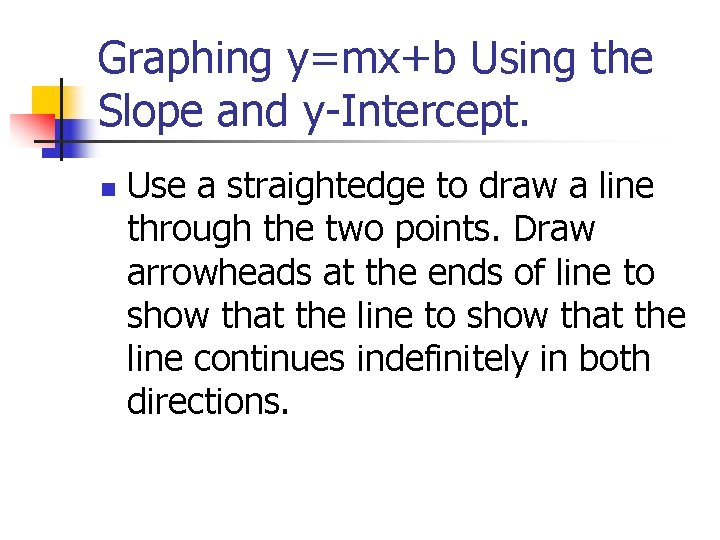Graphing y=mx+b Using the Slope and y-Intercept. n Use a straightedge to draw a line through the two points. Draw arrowheads at the ends of line to show that the line continues indefinitely in both directions.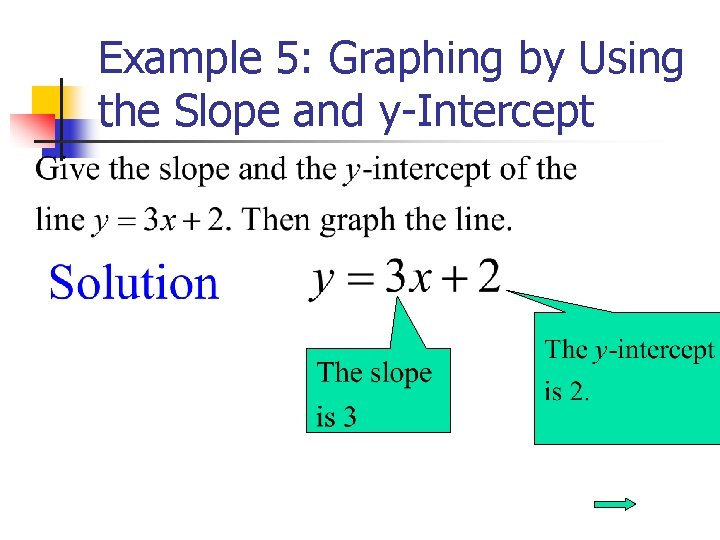Example 5: Graphing by Using the Slope and y-Intercept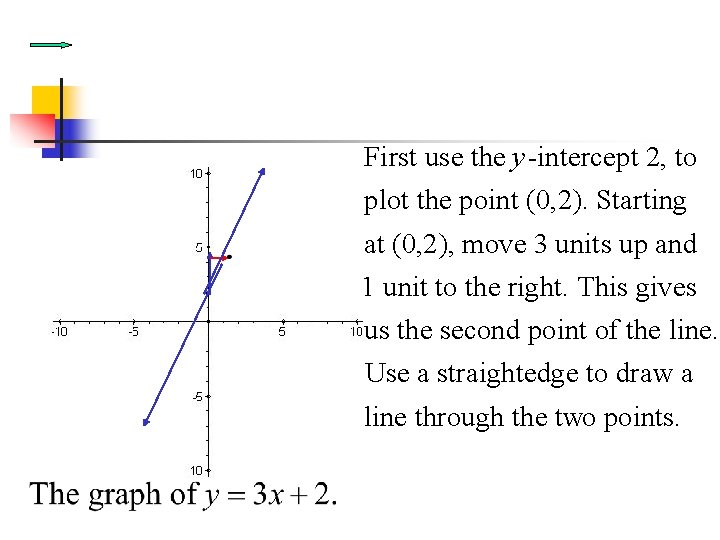First use the y -intercept 2, to plot the point (0, 2). Starting at (0, 2), move 3 units up and 1 unit to the right. This gives us the second point of the line. Use a straightedge to draw a line through the two points.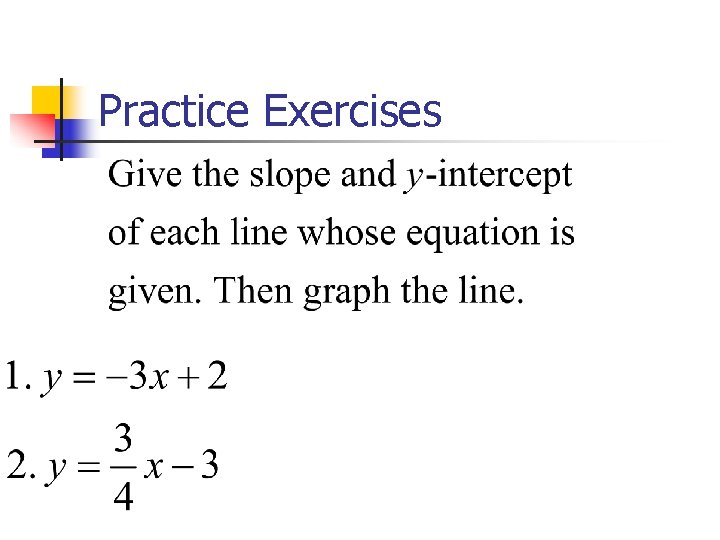Practice Exercises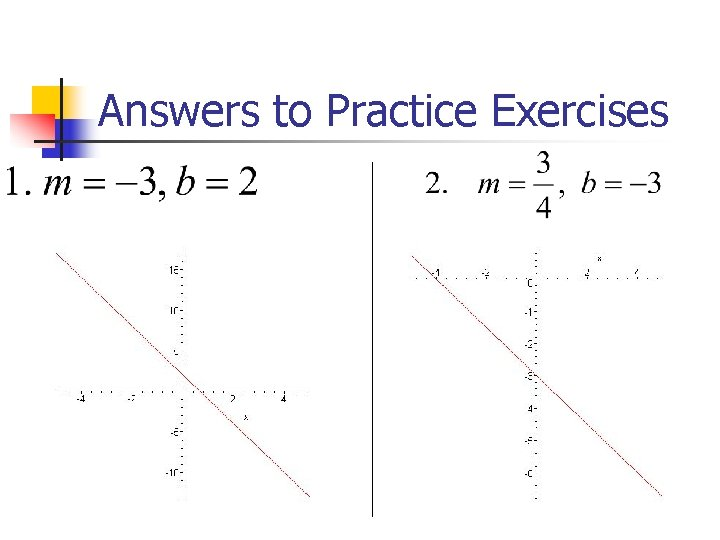Answers to Practice ExercisesEquation of a Horizontal Line Y-intercept is 4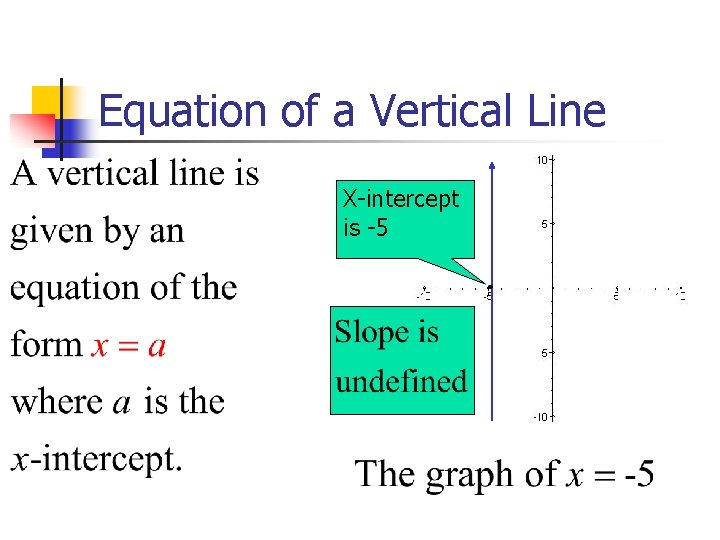Equation of a Vertical Line X-intercept is -5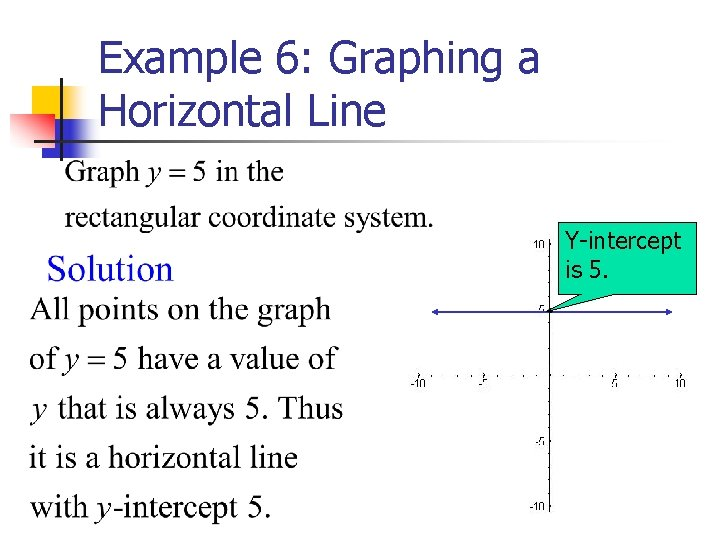Example 6: Graphing a Horizontal Line Y-intercept is 5.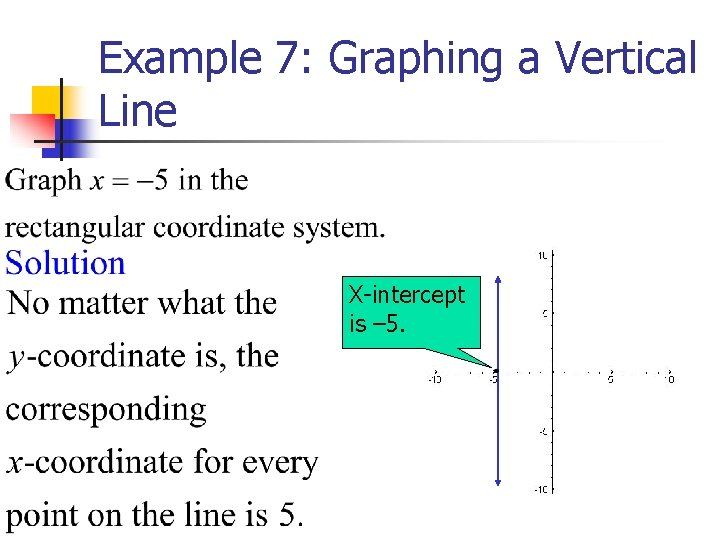Example 7: Graphing a Vertical Line X-intercept is – 5.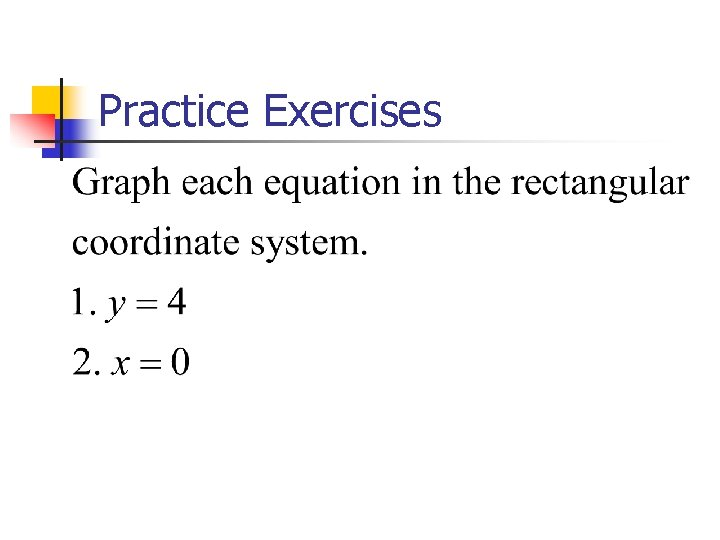Practice ExercisesAnswers to Practice ExercisesGeneral Form of the Equation of a Line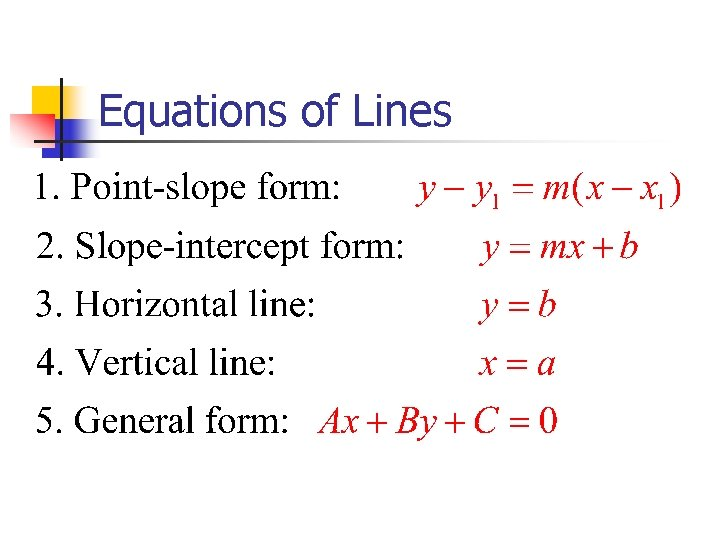Equations of Lines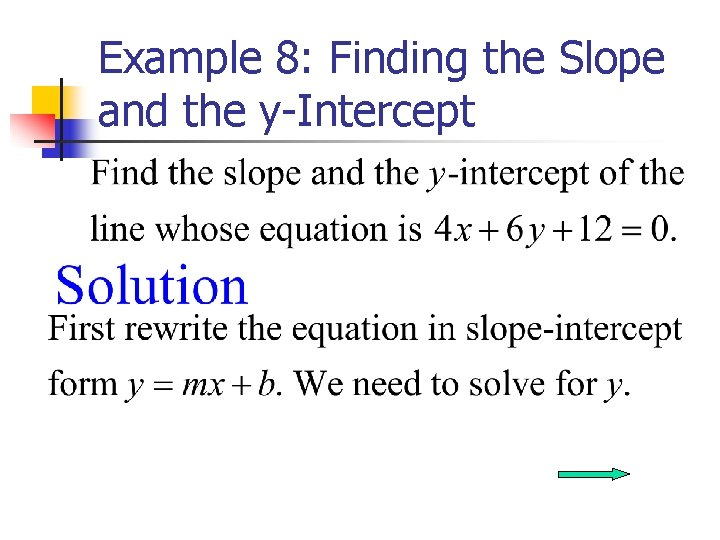Example 8: Finding the Slope and the y-Intercept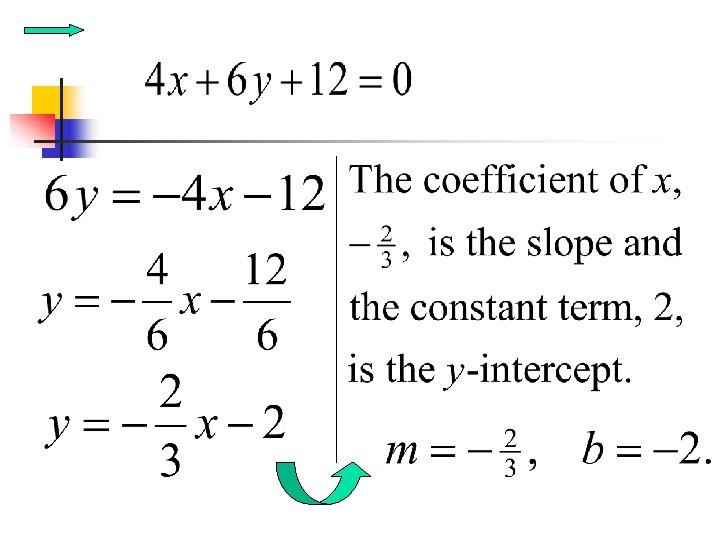Practice ExercisesParallel and Perpendicular Lines n n Parallel lines have the same or equal slopes Perpendicular lines have opposite, reciprocal slopes n Example the following lines are perpendicular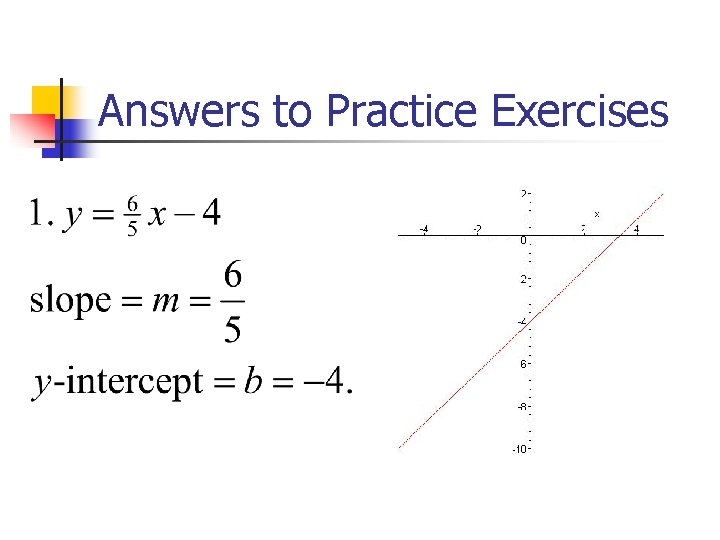Answers to Practice ExercisesAnswers to Practice Exercises